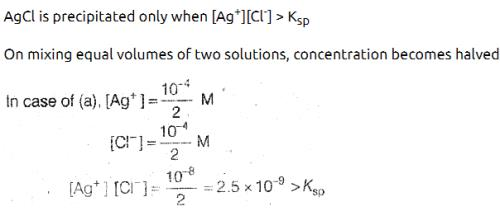Courses

# Test: Ionic Equilibrium (Competition Level)

## 30 Questions MCQ Test Chemistry for JEE | Test: Ionic Equilibrium (Competition Level)

Description
This mock test of Test: Ionic Equilibrium (Competition Level) for Class 11 helps you for every Class 11 entrance exam. This contains 30 Multiple Choice Questions for Class 11 Test: Ionic Equilibrium (Competition Level) (mcq) to study with solutions a complete question bank. The solved questions answers in this Test: Ionic Equilibrium (Competition Level) quiz give you a good mix of easy questions and tough questions. Class 11 students definitely take this Test: Ionic Equilibrium (Competition Level) exercise for a better result in the exam. You can find other Test: Ionic Equilibrium (Competition Level) extra questions, long questions & short questions for Class 11 on EduRev as well by searching above.
QUESTION: 1

### The conjugate acid of NH2- is

Solution:

Conjugate acid of a base is formed by adding H+ to it
NH2- + H+ → NH3

QUESTION: 2

### Out of the following, amphiprotic species are I : HPO32- II OH- III H2PO4- IV HCO3-

Solution:

Amphiprotic : can accept and Release H+
Only H2PO4 & HCO3

QUESTION: 3

### pH of an aqueous solution of NaCl at 85°C should be

Solution:

NaCl Solution : pH is the, pH   of water.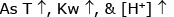pH at 25°C <7

QUESTION: 4

1 c.c. of 0.1N HCl is added to 99 CC solution of NaCl. The pH of the resulting solution will be

Solution:

Volume of resulting solution = 100 ml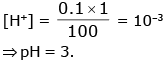QUESTION: 5

10 ml of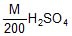is mixed with 40 ml of. The pH of the resulting solution is

Solution: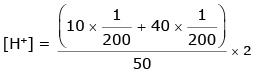[H+] = 10–2
pH = 2.

QUESTION: 6

If pKb for fluoride ion at 25°C is 10.83, the ionisation constant of hydrofluoric acid in water at this temperature is :

Solution:

H+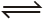H+ + F
pKw = pKa + pKb
[For conjugate Acid-Base]
⇒ pKa = 14 – 10.87 = 3.17
Ka = 6.76 × 10–4

QUESTION: 7

The pH of an aqueous solution of 1.0 M solution of a weak monoprotic acid which is 1% ionised is:

Solution:

Concentration of solution =C=1M
Dissociation is α=0.01
After dissociation, the concentration of H+ ions will be Cα=1×0.01=10−2
pH=−log[H+]=−log(10−2) = +2

QUESTION: 8

If K1 & K2 be first and second ionisation constant of H3PO4 and K1 >> K2 which is incorrect.

Solution: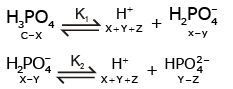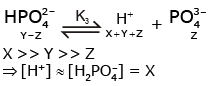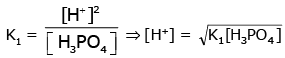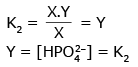QUESTION: 9

The degree of hydrolysis of a salt of weak acid and weak base in it's 0.1 M solution is found to be 50%. If the molarity of the solution is 0.2 M, the percentage hydrolysis of the salt should be

Solution:

% Hydrolysis does notdepend on the conc. in case of “Weak acid + weak base : Salt”

QUESTION: 10

What is the percentage hydrolysis of NaCN in N/80 solution when the dissociation constant for HCN is 1.3 × 10-9 and Kw = 1.0 × 10-14

Solution:

Weak acid + Strong base : Salt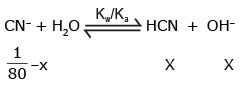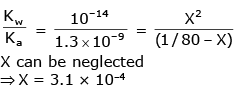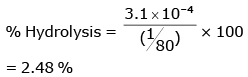QUESTION: 11

The compound whose 0.1 M solution is basic is?

Solution:

Salt of weak acid & strong Base
CH3COONa → CH3COO + Na+ CH3COO+ H2CH3COOH + OH
Basic Solution

QUESTION: 12

Which of the following solution will have pH close to 1.0 ?

Solution:

75mL of M/5HCl + 25mL of M/5 NaOH
Meq of HCl = 15 (∴ Mol. wt. and Eq. wt. of HCl or NaOH are same)
Meq of NaOH = 5 Hence, in solution 10meq of HCl remains in 100mL solution.
So, concentration of HCl in mixture = 10/100M = M/10
In M/10 HCl, [H+] = 10-1 M
pH = -log[H+] = -log10-1 = 1

QUESTION: 13

The ≈ pH of the neutralisation point of 0.1 N ammonium hydroxide with 0.1 N HCl is

Solution:

Salt formed : NH4Cl = 0.1 N
Solution will be slightly acidic due to Hydrolysis

QUESTION: 14

If equilibrium constant of

CH3COOH + H2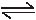CH3COO- + H3O+

Is 1.8 × 10-5, equilibrium constant for

CH3COOH + OH-CH3COO- + H2O is

Solution:

Ka = 1.8 × 10–5
CH3COO + H2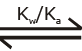CH3COOH + OH-
Given reaction is reverse of above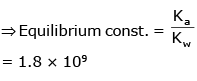QUESTION: 15

If 40 ml of 0.2 M KOH is added to 160 ml of 0.1 M HCOOH [Ka = 2 × 10-4]. The pOH of the resulting solution is

Solution:

m. equivalent of KOH = 8
m. equivalent of HCOOH = 16
Remaining m. eq. (HCOOH) = 8
Formed m. eq. (HCOOK) = 8
⇒ Acidic Buffer
pH = pKa = 4 – log2
= 3.7
pOH = 10.3

QUESTION: 16

A solution with pH 2.0 is more acidic than the one with pH 6.0 by a factor of :

Solution:

[H+]1 = 10–2 ; [H+]2 = 10–6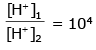QUESTION: 17

The first and second dissociation constants of an acid H2A are 1.0 × 10-5 and 5.0 × 10-10 respectively.

The overall dissociation constant of the acid will be:

Solution: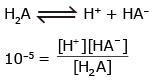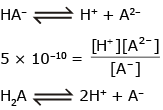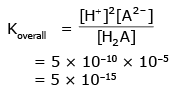QUESTION: 18

An aqueous solution contains 0.01 M RNH2 (Kb= 2 × 10-6) & 10-4 M NaOH.

The concentration of OH- is nearly:

Solution: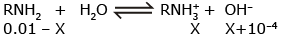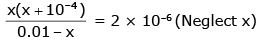x2  +  10–4 x – 2 × 10–8 = 0
x = 10–4
[OH–] = x + 10–4
= 2 × 10–4

QUESTION: 19

What volume of 0.2 M NH4Cl solution should be added to 100 ml of 0.1 M NH4OH solution to produce a buffer solution of pH = 8.7 ?

Given : pKb of NH4OH = 4.7 ; log 2 = 0.3

Solution:

pH = 8.7 ⇒ pOH = 5.3
Basic Buffer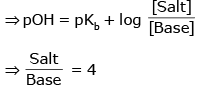If volume of salt = V ml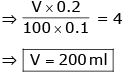QUESTION: 20

The pKa of a weak acid, HA, is 4.80. The pKb of a weak base, BOH, is 4.78. The pH of an aqueous solution of the corresponding salt, BA, will be:

Solution:

Salt of weak acid & weak base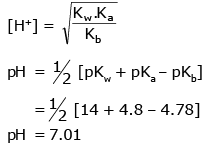QUESTION: 21

The range of most suitable indicator which should be used for titration of X - Na+ (0.1 M, 10 ml) with 0.1 M HCl should be ( Given : kb(X-) = 10-6 )

Solution:

NaX + HCl → NaCl + HX
[HX] at equivalent point = 0.05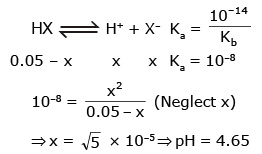Range of Indicator = 3 to 5

QUESTION: 22

How many gm of solid NaOH must be added to 100 ml of a buffer solution which is 0.1 M each w.r.t. Acid HA and salt Na+ A- to make the pH of solution 5.5. Given pKa(HA) = 5 (Use antilog (0.5)= 3.16)

Solution: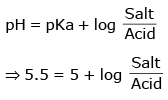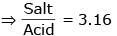Suppose x m. mole NaOH was added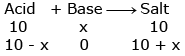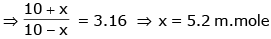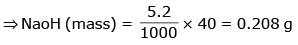QUESTION: 23

The solubility of A2X3 is y mol dm-3. Its solubility product is

Solution: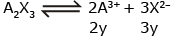Ksp =  (2y)2 (3y)3
Ksp = 108y5

QUESTION: 24

If Ksp for HgSO4 is 6.4 × 10-5, then solubility of this substance in mole per mis

Solution: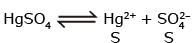Ksp = S2
⇒ 6.4 × 10–5 = S2
⇒ S = 8 × 10–3 mole/L
S = 8 × 10–3 × 103 mole/m3
⇒ S = 8 mole/m3

QUESTION: 25

Which of the following in most soluble in water ?

Solution:

Calculate the solubility ‘s’ for each option, Higher the value of ‘s’ Higher the solubility.

QUESTION: 26

1 M NaCl and 1 M HCl are present in an aqueous solution. The solution is

Solution:

NaCl + HCl : Not the Buffer and Solution is acidic due to HCl.
⇒ pH < 7.

QUESTION: 27

The pKa of a weak acid (HA) is 4.5. The pOH of an aqueous buffered solution of HA in which 50% of the acid is ionized is:

Solution: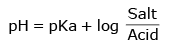50% ionised ⇒ [Salt] = [Acid]
⇒ pH = pKa = 4.5
⇒ pOH = 9.5

QUESTION: 28

The precipitate of CaF2 (Ksp = 1.7 × 10-10) is obtained when equal volumes of the following are mixed

Solution:

For ppt Qsp > Ksp
CaF2→ Ca2++2F
Qsp  = (Ca2+) (F)2
(A) Qsp  = 12.5 × 10–14
(B) Qsp  = 12.5 × 10–10
(C) Qsp  = 12.5 × 10–13
(D) Qsp  = 12.5 × 10–15
Only (B) option will get precipitate.

QUESTION: 29

pH of saturated solution of silver salt of monobasic acid HA is found to be 9.

Find the Ksp of sparingly soluble salt Ag A(s).

Given : Ka(HA) = 10-10

Solution: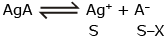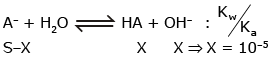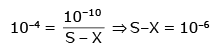Ksp  = S (S–X) = 11 × 10–6 × 10–6
= 1.1 × 10–11

QUESTION: 30

When equal volumes of the following solutions are mixed, precipitation of AgCl (Ksp = 1.8 × 10-10) will occur only with:

Solution: